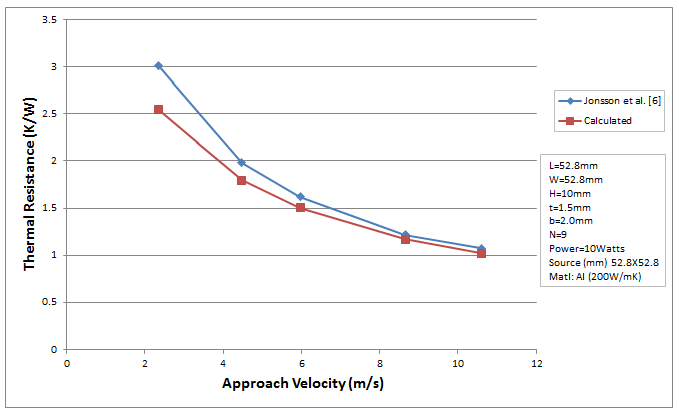18 Apr

The Heat Sink Calculator uses a combination of semi-empirically derived equations to calculate the heat transfer and pressure drop due to forced convection. Equations for laminar  and turbulent  flow are combined to form a single equation that is suitable for calculating the convection coefficient for laminar, transition to turbulent and turbulent flows through the heat sink. Similarly the pressure drop is calculated using laminar and turbulent flow correlations developed by Shah and London  and Zhi-Qing  respectively.

The results obtained from the Heat Sink Calculator have been compared with experimental data that span the laminar and turbulent flow regimes. The graphs below show experimental and calculated data for heat sinks undergoing forced convection. The experimental data are obtained from work done by Saini et al.  and Jonsson et al. .

The experiments conducted by Jonsson used a heat sink whose external sides were exposed to the flow of air. In order to compare the results from the Heat Sink Calculator which models a fully ducted heat sink with no airflow over the external side surfaces the thermal resistance values from Jonsson were modified using the following equation.$Latex formula$

where:$Latex formula$      adjusted thermal resistance$Latex formula$      thermal resistance measurement from$Latex formula$         heat transfer area of the heat sink from$Latex formula$   area of the external heat sink sides exposed to air flow inThe calculation results are in good agreement with the experimental results, within 8% RMS for thermal resistance and 10% RMS for the pressure drop.

References:
 P. Teertstra, M. Yovanovich, J.R. Culham, “Analytical Forced Convection Modeling of Plate Fin Heat Sinks”, in: Proceedings of the 15th IEEE Semi-Thermal Symposium, 1999, pp. 34-41

 V. Gnielinski, “New Equations for Heat and Mass Transfer in Turbulent Pipe and Channel Flow”, in: International Chemical Engineer 16 (2), 1976, pp. 359-368

 R.K. Shah, A.L. London, “Laminar Flow Forced Convection in Ducts”, Academic Press New York, 1978

 M.S. Bhatti, R.K. Shah, “Handbook of Single-Phase Convective Heat Transfer”, Wiley-Interscience, New York, 1987 Chap. 4

 M. Saini, and E.L. Webb,  “Validation of Models for Air Cooled Plane Fin Heat Sinks used in Computer
Cooling”, in: Thermal and Thermomechanical Phenomena in Electronic Systems, 2002. ITHERM 2002. The Eighth Intersociety Conference 2002, pp. 243-250

  H. Jonsson, B. Moshfegh, “Modeling of the Thermal and Hydraulic Performance of Plate Fin, Strip Fin, and Pin Fin Heat Sinks – Inﬂuence of Flow Bypass”, in: IEEE Transactions on Components and Packaging Technologies, Vol. 24, No. 2, 2001, pp. 142-149